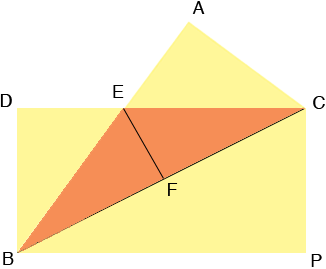SEARCH HOMEMath Central Quandaries & QueriesQuestion from Amit, a teacher: A rectangular sheet of a paper with dimensions a and b is folded along its diagonal. What is the area of the overlapped region?Amit,

I folded the sheet of paper CDBP along the diagonal CB so that P moved to A. then drew a line segment EF to meet BC in a right angle at F. Let |CA| = a, |AB| = b and then |BC| = c where c2 = a2 + b2 by Pythagoras' Theorem.By the symmetry F is the midpoint of BC. Let |EF| = h, the height of triangle CEB.

Triangles ABC and EBF are similar.

Can you complete the problem now?
HarleyMath Central is supported by the University of Regina and The Pacific Institute for the Mathematical Sciences.